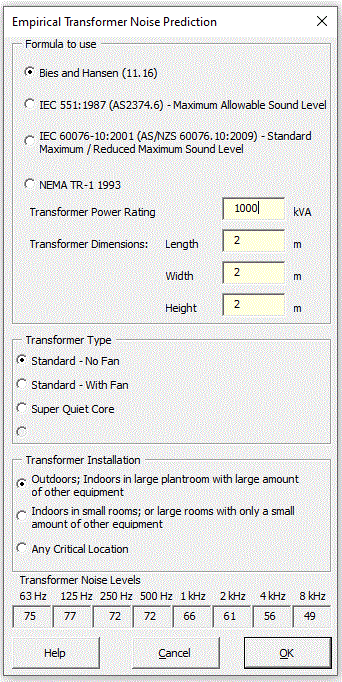### Strutt Help

Empirical Transformer Noise Prediction    1/1

Strutt|Input Data|Empirical|Transformer inserts sound power levels into the active row of the worksheet.The sound power level of transformers can be calculated using formulae from Bies and Hansen or IEC 551:1987.

Bies and Hansen calculate the total sound power level using the equation:

L_W = N_R + 10log_10 S + C, (dB re 10-12 W)

Where,
N_R is the NEMA sound level rating, which is the average sound pressure level measured around the transformer at a distance of 0.35 m. Values of NR based on transformer power (KVA) and transformer type are sourced from Table 11.28 Bies and Hansen.
S is the surface area (m2) of the four transformer walls (excluding the top).
C is the correction term, values are as listed in Table 11.27 Bies and Hansen. The value of C depends on the installation location.
Octave band sound power levels are then calculated by subtracting the values in Table 11.26 Bies and Hansen.

IEC 551:1987 calculates the overall A-weighted sound power level by:

L_(WA) = 74 + 14log_10(MVA), where MVA is larger than 0.2;
L_(WA) = 64, where MVA is less than 0.2

The installation location and octave band sound power level are calculated as for Bies and Hansen.

IEC 60076-10:2001 calculates the overall A-weighted Standard Maximum sound power level by:

L_(WA) = 66 + 14log_10(MVA), where MVA is larger than 0.2;
L_(WA) = 56, where MVA is less than or equal to 0.2

The Reduced Maximum sound power level is calculated by:

L_(WA) = 58 + 14log_10(MVA), where MVA is larger than 0.75;
L_(WA) = 56, where MVA is less than or equal to 0.75

The installation location and octave band sound power level are calculated as for Bies and Hansen.

References:

• Bies, D., Hansen, C., Engineering Noise Control pp554-556
• AS2374.6 (IEC 551:1987) pp22-23
• AS 60076.10:2009 Annex ZA4 / IEC 60076-10:2001